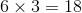# ACT Math : How to find the length of the side of an equilateral triangle

## Example Questions

### Example Question #1 : How To Find The Length Of The Side Of An Equilateral Triangle

The height of an equilateral triangle is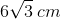. Find the perimeter.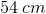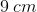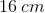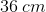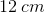Explanation:

From the given information, we can draw the following picture: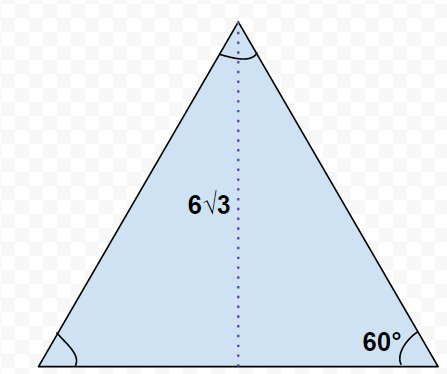Since this is an equilateral triangle, all three of its angles will be congruent. All three of its sides will also be congruent.

The dotted line, representing the height, bisects not only the base of the triangle but also the apical angle. This means that the two smaller right triangles created by the dotted line have bases that are half the side length of the equilateral triangle with apical angle measures ofThis creates two smaller 30/60/90 special right triangles. This problem can be solved in one of two ways:

1. You can use the derived side ratio for 30/60/90 triangles,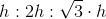, to solve for the length of one of the equilateral triangle's sides.

OR

2. You can use trig functions to solve for the length of one of the equilateral triangle's sides.

Using trig functions, we can useas our angle of interest, with the length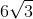and the hypotenuse being our mystery side.

Going back to SOH CAH TOA, we can determine that we will be using sine because we have the information available for the side opposite ("O") of our angle of interest and are looking for the hypotenuse ("H"). This means we will be using the "SOH" part of SOH CAH TOA—sine.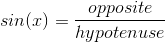Substituting in the problem's values, we can solve for the length of the hypotenuse of one of the two smaller triangles, which is the length of one side of the equilateral triangle: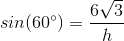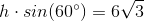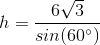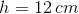Remember that the problem asks for the triangle's perimeter. That means that we need to multiply the length of one of its edges by three to find the final answer: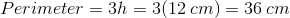### Example Question #2 : How To Find The Length Of The Side Of An Equilateral Triangle

If an equilateral triangle has a height of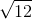and an area of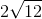, what is the measure of one of its sides?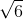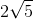Explanation:

For any triangle,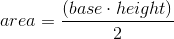.

We know our height isand our area is.

Therefore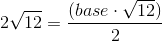so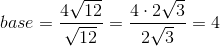.

### Example Question #3 : How To Find The Length Of The Side Of An Equilateral Triangle

An equilateral triangle has an area of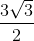and a height of. What is the length of one of its sides?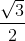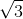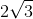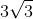Explanation:

Since this triangle is equilateral, all of the sides (including the base) are equal.

Use the formula for area of a triangle to solve for the base: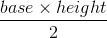In this case: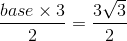So the base is equal to### Example Question #4 : How To Find The Length Of The Side Of An Equilateral Triangle

An equilateral triangle has a height of. What is the perimeter of this triangle?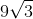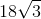Explanation:

Since this is an equilateral triangle, all of the sides and all of the angles would be equal (60 degrees each since the 3 angles must add up to 180 degrees).

1. The height splits the triangle's base in half and creates two right triangles. Create expressions for the length of the two unknown sides in this new triangle:

The value we are looking for is the side length so we'll make thatThe base of the new triangle is half the length of a side so we'll make that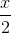2. Use the Pythagorean Theorem to find the value ofor the side length:In this case: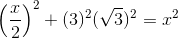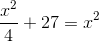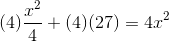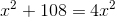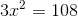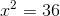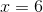3. Multiply the side length you found by 3 to get the perimeter: